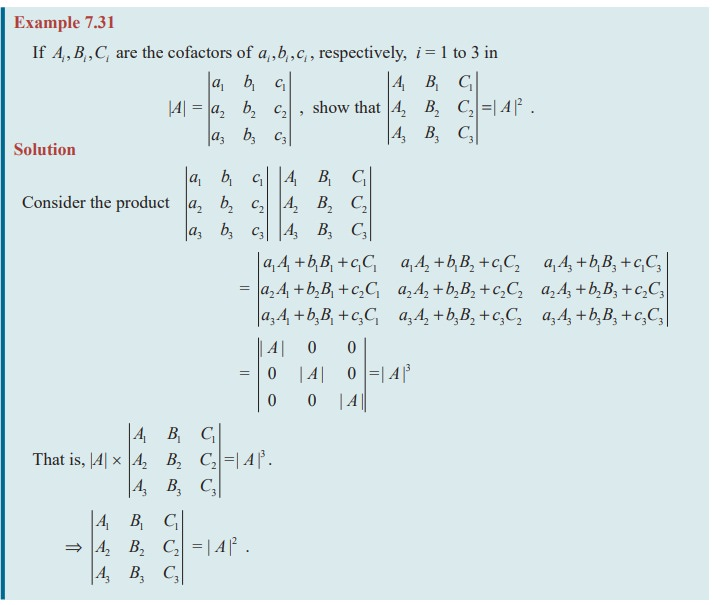Home | | Maths 11th std | Relation between a Determinant and its Cofactor Determinant

# Relation between a Determinant and its Cofactor Determinant

Note that the sum of the product of elements of any row (or column) with their corresponding cofactors is the value of the determinant.

Relation between a Determinant and its Cofactor DeterminantLet A1, B1, C1.... be the cofactors of a1, b1, c1 ... in | A |.Similarly, | A |= a2 A2 + b2 B2 + c2 C2 And  | A |= a3 A3 + b3 B3 + c3C3

Note that the sum of the product of elements of any row (or column) with their corresponding cofactors is the value of the determinant.= − a1 (b1c3b3 c1 ) + b1 (a1c3a3 c1 ) − c1 (a1b3a3b1 )

= a1b1c3 + a1b3 c1 + a1b1c3a3b1c1a1b3 c1 + a3 b1c1 = 0

Similarly we get

a1 A3 + b1 B3 + c1C3  = 0 ;  a2 A1 + b2 B1 + c2 C1 = 0 ;

a2 A3 + b2 B3 + c2 C3= 0 ;  a3 A1 + b3 B1 + c3C1 = 0 and a3 A2 + b3 B2 + c3C2 = 0 .

Note 7.12

If elements of a row (or column) are multiplied with corresponding cofactors of any other row (or column) then their sum is zero.Study Material, Lecturing Notes, Assignment, Reference, Wiki description explanation, brief detail
11th Mathematics : UNIT 7 : Matrices and Determinants : Relation between a Determinant and its Cofactor Determinant |